# Difference between revisions of "Cartesian-closed category"

A category $\mathfrak{C}$ such that the following axioms are satisfied:

A1) there exists a terminal object $\mathbf{1}$;

A2) for any pair $A,B$ of objects of $\mathfrak{C}$ there exist a product $A \times B$ and given projections $\mathrm{pr}_1 : A \times B \rightarrow A$, $\mathrm{pr}_2 : A \times B \rightarrow B$;

A3) for any pair $A,B$ of objects of $\mathfrak{C}$ there exist an object $A^B$ and an evaluation arrow $\mathrm{ev} : A^B \times B \rightarrow A$ such that for any arrow $F : C \times A \rightarrow B$ there is a unique arrow $[f] : C \rightarrow A^B$ with $\mathrm{ev}\circ ([f]\times \mathrm{id}_A) = f$.

These conditions are equivalent to the following: $\mathfrak{C}$ is a category with given products such that the functors $$\mathfrak{C} \rightarrow \mathbf{1}\,\ \ c \mapsto 0\,;$$ $$\mathfrak{C} \rightarrow \mathfrak{C} \times \mathfrak{C}\,\ \ c \mapsto \langle c,c \rangle \,;$$ $$\mathfrak{C} \rightarrow \mathfrak{C}\,\ \ c \mapsto c \times b$$ have each a specified right-adjoint, written respectively as: $$0 \mapsto t\,;$$ $$\langle a,b \rangle \mapsto a \times b\,;$$ $$c \mapsto c^b \ .$$

Some examples of Cartesian-closed categories are:

E1) any Heyting algebra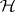;

E2) the category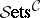for any small category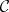with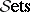the category of (small) sets — in particularitself;

E3) the category of sheaves over a topological space, and more generally a (Grothendieck) topos;

E4) any elementary topos;

E5) the category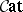of all (small) categories;

E6) the category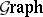of graphs and their homomorphisms;

E7) the category-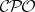of-CPOs.

These definitions can all be put into a purely equational form.

How to Cite This Entry:
Cartesian-closed category. Encyclopedia of Mathematics. URL: http://encyclopediaofmath.org/index.php?title=Cartesian-closed_category&oldid=42620
This article was adapted from an original article by M. Eytan (originator), which appeared in Encyclopedia of Mathematics - ISBN 1402006098. See original article